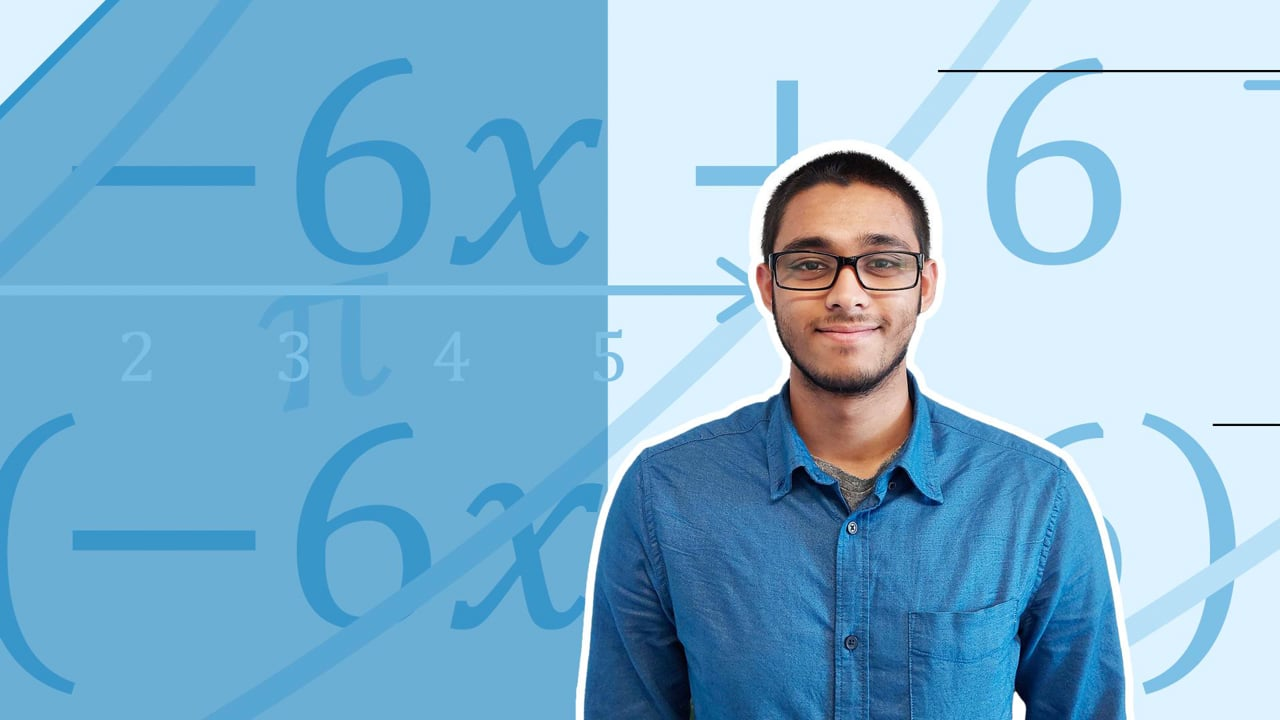Chapter overviewMaths

Exam board

AQA

Number

Algebra

Graphs

Ratio proportion and rates of change

Shapes and area

Angles and geometry

Trigonometry

Probability

Statistics

Maths

# The quadratic formula - Higher0%

Summary

## ​​In a nutshell

A quadratic equation in the form $ax^2+bx+c=0$ can be solved using the quadratic formula. Sometimes, it is necessary to use the quadratic formula to solve a quadratic rather than factorising, as not all quadratics can be factorised easily.

$x= \dfrac{-b \pm \sqrt{b^2-4ac}} {2a}$​​

Where $a,b$ and $c$ are the co-efficients from the quadratic equation in the form

$ax^2+bx+c=0$

Substitute the values of $a, b$ and $c$ from the quadratic equation into the quadratic formula, and calculate the solutions for $x$.

### Example 1

Use the quadratic formula to solve

$2x^2+7x+3=0$​​

From the quadratic equation, $a=2, b=7$ and $c=3$. Substitute the numbers into the quadratic formula.

$x= \dfrac{-b \pm \sqrt{b^2-4ac}} {2a}$​​

$x= \dfrac {-7 \pm \sqrt {7^2-4\times2\times3}} {2 \times 2}\\$​​

Work out the value inside the square root.

$x= \dfrac {-7 \pm \sqrt {25}} {4}$​​

$x= \dfrac {-7 \pm 5} {4}\\$​​

The $\pm$ symbol means you have to do plus or minus, so there will be two calculations

$x = \dfrac {-7+5} 4 \space or \space x= \dfrac {-7-5} 4$​​

$\underline{x=-\frac 1 2} \space or \space \underline{x=-3}$

## Want to find out more? Check out these other lessons!

FAQs

• Question: What are the different ways to solve a quadratic?

Answer: A quadratic equation can be solved by factorising, using the quadratic formula or using complete the square.

• Question: What is the quadratic formula used for?

Answer: The quadratic formula calculates the roots or solutions to a quadratic equation. The formula can be used as an alternative to factorising.

• Question: When do you use the quadratic formula to solve a quadratic?

Answer: Sometimes, it is necessary to use the quadratic formula to solve a quadratic rather than factorising, as not all quadratics can be factorised easily.

Theory

Exercises